﻿ Ultimate Symmetry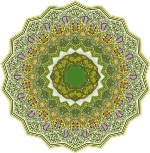## Complex-Time Geometry and the Five Regular Polyhedra

In accordance with the four classical elements and their Quintessence, there are exactly five geometric solids whose faces are regular and identical polygons meeting at equal three-dimensional angles. These five regular polyhedra are the only solid shapes with this sort of complete symmetry. Many philosophers wondered why there cannot be more, or fewer, so perfectly symmetrical shapes. They are usually called Platonic Solids, because Plato composed a complete theory of the cosmos based on their definite shapes, associating them with the four classical elements and the Quintessence. It is worth mentioning here that, according to the genuinely-complex time-time geometry, as we have introduced in section II.3.4 above, there are exactly five fundamental interactions or fields: magnetic, strong, weak, electric and gravity, corresponding to the dimensions: 0T, 1T, 3T, 5T and 7T, respectively.

As summarized in table 4, the names of these polyhedra reflect the number of faces that each one possesses, and these same names may be also used to describe other three-dimensional solids that have the same number of faces. At least some of these solids were known by Pythagoras, or even before, but according to Euclid, the Octahedron and Icosahedron were first discussed by the Theaetetus (c. 417–369 BC). Plato was greatly impressed by these five definite shapes that constitute the only perfectly symmetrical arrangements of a set of non-planar points in space, so he expounded a complete theory of the cosmos based explicitly on these five solids. In his dialogue Timaeus, he associated four of these polyhedrons with the four basic elements: assigning the Tetrahedron to the element Fire, because of its sharp points and edges, as shown in Figure IV.4, while the Cube corresponded to Earth because of its four-square regularity, as shown in Figure IV.5, and the Octahedron to Air since its minuscule components are so smooth that one can barely feel it, as shown in Figure IV.6, and finally the Icosahedron, shown in Figure IV.7, to Water that flows out of one’s hand when picked up, as if it is made of tiny little balls. The fifth solid, the Dodecahedron, as shown in Figure IV.8, with its twelve pentagonal faces, was assigned to the Heavens with its twelve zodiac constellations, since also it is so different from the other polyhedra, in virtue of its pentagonal faces. Timaeus contains a very detailed discussion of virtually all aspects of physical existence, including biology, cosmology, geography, chemistry, physics, psychological perceptions, all expressed in terms of these four basic elements and their transmutations from one into another by means of the constituent triangles being broken apart and re-assembled into other forms.

With these hypothetical relations, Plato developed a theory of the Universe based on the five regular polyhedra, hence they became known as the Platonic solids, though even this correspondence was defined earlier by the philosopher Empedocles. Theaetetus gave a mathematical description of all five and may have been responsible for the first known proof that no other convex regular polyhedra exist.

After two millenniums, the astronomer Johannes Kepler (1571–1630) resuscitated the idea of using the regular polyhedra to explain the geometry of the Universe in his first model of the cosmos. He attempted to explain the motion of the planets based on these solids, where he concluded that the distances of the orbits, which he assumed were circular, were related to the regular polyhedra in their proportions. In Mysterium Cosmographicum, published in 1596, Kepler proposed a model of the Solar System in which the five solids were set inside one another and separated by a series of inscribed and circumscribed spheres. The solids were ordered with the innermost being the Octahedron, followed by the Icosahedron, Dodecahedron, Tetrahedron, and finally the cube, thereby dictating the structure of the solar system and the distance relationships between the planets by the regular polyhedra. Later in his life, after his friend the great astronomer Tycho Brahe bequeathed to him an enormous collection of astronomical observations, Kepler finally reason to the conclusion that this model of planetary motion was mistaken, and that in fact planets moved around the sun in ellipses, not circles.

A regular polygon is one for which all the edges are the same length, and all the angles between the edges are also the same. Only triangles, squares and pentagons can contribute to make a regular polyhedra, because the angles of all other polygons are too large to make solids without leaving out some extra space that cannot be filled by the the same polygon. With an hexagon, for example, we can have either two sides meeting at each vertex, or three. In the first case we cannot form a regular solid, and in the second case we will get an infinite tiling of the plane. Any regular polygon with more than six sides will not work either, since the only way we can combine them is to have two meeting per vertex, because three would overlap even on a plane.

In contrast, with pentagons, we can have three of them meeting per vertex, without any extra space. This is also the case with squares, since we can also have only three of them meeting per vertex, while four squares will produce an infinite tiling of the plane. In the case of triangles, we have three possibilities, since we can have either three, four or five triangles meeting per vertex. If we try to fit six of them, we would give an infinite tiling of the plane.

### The Tetrahedron

A regular Tetrahedron is one in which all four faces are equilateral triangles. It is the simplest of all the ordinary convex polyhedra and the only one that has fewer than 5 faces. It has four vertices, six edges and four triangular faces, and that’s why it is also known as a triangular pyramid. For any Tetrahedron there exists a sphere, called the circumsphere, on which all four vertices lie, and another sphere, called the insphere, tangent to its faces. Like all convex polyhedra, a Tetrahedron can be folded from a single sheet of paper.

The regular Tetrahedron is self-dual, which means that its dual is itself, and the compound figure comprising two such dual tetrahedra form a stellated Octahedron or stella octangula, which means “eight-pointed star”. Unlike to what Plato suggested, this distinctive property of Tetrahedron makes it correspond to Quintessence, in addition to its being the simplest of them all. Just like the four elements, two of the other four solid are the duals of the other two, as we shall see further below.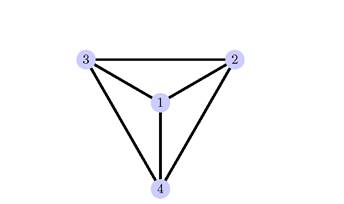Figure IV.4: The Tetrahedron has four faces, which are equilateral triangles, and four vertices, and six edges. It is the dual of itself, and it is also called a triangular pyramid.

### The Hexahedron

The Hexahedron, which is the Cube, has six square faces, eight vertices, and twelve edges. A Hexahedron is any polyhedron with six faces, but the Cube is a regular Hexahedron with all its faces square, and three squares around each vertex. The cube is the only regular Hexahedron. The cube is also a square parallelepiped, an equilateral cuboid and a right rhombohedron. It is also a regular square prism in three orientations, and a trigonal trapezohedron in four orientations. The cube is dual to the Octahedron. It has cubical or octahedral symmetry. The cube is the only convex polyhedron whose faces are all squares.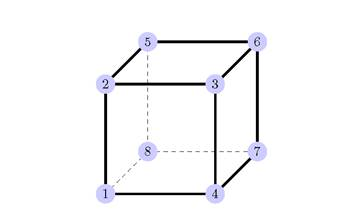Figure IV.5: The Hexahedron, or the Cube, has six square faces, eight vertices, and twelve edges, and its the dual of the Octahedron.TC "4.5 " \f f

Plato suggested that the Cube corresponds to the element Earth, because of its four-square regularity, but this same property makes it correspond to Water, whose complex time-time structure is (c, c), rather than Earth (0, 0), because the physical world, described by (c, v), is made from Water that is the real vacuum super-fluid as we explained in Chapter II. Also, since the dual of the Hexahedron is the Octahedron, that should corresponds with Air, which is the super-gas state (c, 0) that is the vacuum of the psychical world, and that is why these two solids are mutually orthogonal, just like the two arrows of time which are the folds of super-symmetry, as we explained in Chapter II.

### The Octahedron

The Octahedron has eight triangular faces, six vertices, and twelve edges. A regular Octahedron consists of eight equilateral triangles, with four of those triangles meeting at each vertex. It is in fact the only one of the regular polyhedra to have an even number of faces meeting at a single vertex. Various minerals have been found in the form of octahedral crystals, including diamond, alum and fluorite. Interestingly, there are two different vertex angles: at any vertex, the angle between adjacent edges is sixty degrees, whereas the angle between opposite edges is ninety degrees.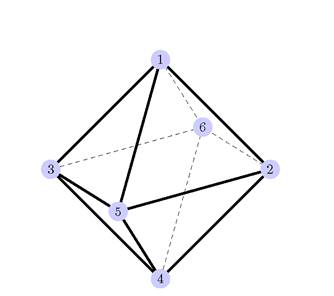Figure IV.6: The Octahedron, eight triangular faces, six vertices, and twelve edges, and its the dual of the Hexahedron. TC "4.6 " \f f

As we mentioned above, Plato suggested that the Octahedron corresponds to the element Air, since its minuscule components are so smooth that one can barely feel it. This goes well in accordance with the complex-geometry of time as we noted above, since the Octahedron is the dual of Hexahedron and they both form two mutually orthogonal arrows of time which are the folds of super-symmetry, as we explained in Chapter II.

### The Icosahedron

The Icosahedron has twenty triangular faces, twelve vertices, and thirty edges. A regular Icosahedron consists of twenty equilateral triangles, with five of those triangles meeting at each vertex. The Icosahedron is the only one of the regular polyhedra to have a dihedral angle with a magnitude greater than one hundred and twenty degrees. Like the Octahedron, the Icosahedron has two different vertex angles: at any vertex, the angle between adjacent edges is sixty degrees, whereas the angle between non-adjacent edges is one hundred and eight degrees.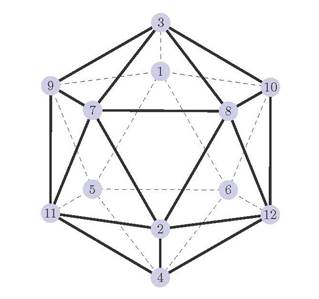Figure IV.7: The Icosahedron has twenty triangular faces, twelve vertices, and thirty edges, and its dual is the Dodecahedron.

Plato suggested that Icosahedron corresponds to Water that flows out of one’s hand when picked up, but as we showed above, Water should be assigned with the Cube that resembles the physical world which is the excitations of the super-fluid vacuum (c, 0) that is the element Water, and has nothing to do with water as the chemical compound. The Icosahedron should correspond with Earth, as the absolute mass state (0, 0), since its dual is the Dodecahedron that corresponds to Fire as the absolute energy state (c, c).

### The Dodecahedron

The Dodecahedron has twelve pentagonal faces, twenty vertices, and thirty edges. A regular Dodecahedron consists of twelve regular pentagons, with three of those pentagons meeting at each vertex. The pyritohedron, a common crystal form in pyrite, is an irregular pentagonal Dodecahedron, having the same topology as the regular one but pyritohedral symmetry while the tetartoid has tetrahedral symmetry.Figure IV.8: The Dodecahedron has twelve pentagonal faces, twenty vertices, and thirty edges, and its dual is the Icosahedron. TC "4.8 " \f f

With its twelve pentagonal faces, Plato assigned the Dodecahedron to the Heavens with its twelve zodiac constellations. This agrees very well with the complex-time structure, but the Heavens is the element Fire (c, c) and the not the Quintessence that corresponds to the Tetrahedron as we mentioned above. As we also said, the dual of the Dodecahedron is the Icosahedron which corresponds to the Earth state (0, 0).

If the five regular polyhedra are built with same volume, the regular Dodecahedron has the shortest edges, and the distance between the vertices on the same face not connected by an edge equals the edge length multiplied the golden ration.

The symmetry, structural integrity, and beauty of these solids have inspired architects, artists, and artisans from ancient Egypt to the present. Euclid devoted the last book of the Elements to the regular polyhedra, where he provided the first known proof that exactly five regular polyhedra exist. It is natural to wonder why there should be exactly five regular polyhedra, and whether there might conceivably be one that simply hasn’t been discovered yet. However, it is not difficult to show that there must be five, and that there cannot be more than five. Thus Plato concluded that they must be the fundamental building blocks of nature.

The regularity of the regular polyhedra means that they are all highly symmetrical. For each Platonic solid, it is possible to construct a circumscribed sphere or circumsphere that completely encloses the Platonic solid, and for which all of the vertices of the Platonic solid lie on the surface of the sphere, a mid-sphere that is tangent to each of the edges of the Platonic solid, and an inscribed sphere or in-sphere that is completely enclosed by the Platonic solid, and that is tangent to each of its faces. For each of the regular polyhedra, these three spheres are concentric. The radii of the spheres are called the circum-radius, the mid-radius, and the in-radius respectively.

The symmetry groups of the regular polyhedra are a special class of three-dimensional point groups known as polyhedral groups. The high degree of symmetry of the regular polyhedra can be interpreted in a number of ways. Most importantly, the vertices of each solid are all equivalent under the action of the symmetry group, as are the edges and faces. One says the action of the symmetry group is transitive on the vertices, edges, and faces. In fact, this is another way of defining regularity of a polyhedron: a polyhedron is regular if and only if it is vertex-uniform, edge-uniform, and face-uniform. There are only three symmetry groups associated with the regular polyhedra rather than five, since the symmetry group of any polyhedron coincides with that of its dual. This is easily seen by examining the construction of the dual polyhedron. Any symmetry of the original must be a symmetry of the dual and vice versa. The three polyhedral groups are: the tetrahedral group, the octahedral group, which is also the symmetry group of the cube, and the icosahedral group, which is also the symmetry group of the Dodecahedron. The orders of the proper rotation groups are 12, 24, and 60 respectively; precisely twice the number of edges in the respective polyhedra. The orders of the full symmetry groups are twice as much again (24, 48, and 120). All regular polyhedra except the Tetrahedron are centrally symmetric, meaning they are preserved under reflection through the origin.

### Message from the Author:I have no doubt that this is the most significant discovery in the history of mathematics, physics and philosophy, ever!

This unique understanding of geometry will cause a paradigm shift in our knowledge of the fundamental nature of the cosmos and its corporeal and incorporeal structures.

Enjoy reading... , all the Best !

Mohamed bin Ali Haj Yousef

Check this detailed video presentation on "Deriving the Principles of Special, General and Quantum Relativity Based on the Single Monad Model Cosmos and Duality of Time Theory".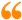The science of Time is a noble science, that reveals the secret of Eternity. Only the Elites of Sages may ever come to know this secret. It is called the First Age, or the Age of ages, from which time is emerging.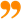Ibn al-Arabi [The Meccan Revelations: Volume I, page 156. - Trns. Mohamed Haj Yousef]

### Ibn al-Arabi Website:### The Meccan Revelations:### The Sun from the West:## Social Sharing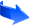Like Our Facebook Page:Like Our Facebook Page:My soul is from elsewhere, I'm sure of that, and I intend to end up there.